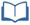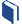# Which Model Makes Sense?

Estimated Time: 75-90 minutes

###Lesson Objectives:

• Students will gather and analyze data to determine a model of best fit (linear, quadratic or exponential).
• Students will apply their understanding of residuals and the correlation coefficient to justify the model.

###Resources for this lesson:

Before you begin, download your Algebra II Journalfor Which Model Makes Sense? You will be completing activities in the journal throughout this lesson.

Visit this section on each page to access information about key terms and other resources to help facilitate the lesson.

> Glossary> Calculator Resources> Teacher Resources: Instructional NotesKey Common Core State Standards:

S.ID.6 Represent data on two quantitative variables on a scatter plot and describe how the variables are related.

1. Fit a function to the data; use functions fitted to data to solve problems in the context of the data.
2. Informally assess the fit of a function by plotting and analyzing residuals.
3. Fit a linear function for a scatter plot that suggests a linear association.

Supporting Common Core State Standards:

F.IF.4 For a function that models a relationship between two quantities, interpret key features of graphs and tables in terms of the quantities, and sketch graphs showing key features given a verbal description of the relationship.

F.LE.1 Distinguish between situations that can be modeled with linear functions and with exponential functions.

Standards for Mathematical Practices:

MP.1 Make sense of problems and persevere in solving them.
MP.2 Reason abstractly and quantitatively.
MP.3 Construct viable arguments and critique the reasoning of others.
MP.4 Model with mathematics.
MP.5 Use appropriate tools strategically.
MP.6 Attend to precision.

Next >